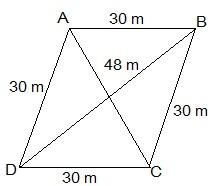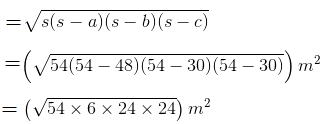Guru

# A rhombus shaped field has green grass for 18 cows to graze. If each side of the rhombus is 30 m and its longer diagonal is 48 m, how much area of grass field will each cow be getting? Q.5

• 0

Please guide me the best way for solving the question of class 9th math of Heron’s Formula chapter of exercise 12.2  of math of question no. 5 What is the best way for solving this question, please guide me A rhombus shaped field has green grass for 18 cows to graze. If each side of the rhombus is 30 m and its longer diagonal is 48 m, how much area of grass field will each cow be getting?

Share

1. Draw a rhombus-shaped field first with the vertices as ABCD. The diagonal AC divides the rhombus into two congruent triangles which are having equal areas. The diagram is as follows.Consider the triangle BCD,

Its semi-perimeter = (48 + 30 + 30)/2 m = 54 m

Using Heron’s formula,

Area of the ΔBCD == 432 m2

∴ Area of field = 2 × area of the ΔBCD = (2 × 432) m2 = 864 m2

Thus, the area of the grass field that each cow will be getting = (864/18) m2 = 48 m2

• 0